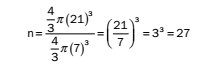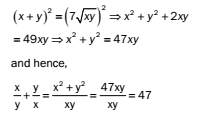# RRB ALP 2018 Practice Test Papers | Arithmetic Questions (Day-22)

Dear Aspirants, Here we have given the Important RRB ALP & Technicians Exam 2018 Practice Test Papers. Candidates those who are preparing for RRB ALP 2018 can practice these Arithmetic Questions to get more confidence to Crack RRB 2018 Examination.

[WpProQuiz 1803]

Click “Start Quiz” to attend these Questions and view Explanation

1. The average of 30 numbers is 24. If two numbers namely 36 and 40 are discarded, what is the average of the remaining numbers?

(A) 23

(B) 25

(C) 26

(D) 27

1. Find the difference between the compound interest and simple interest at 10% p.a. for 2 years on a principal of Rs.1600?

(A) 15

(B) 16

(C) 20

(D) 18

1. The expenditures of A, B and C are in the ratio 3: 8: 11 and the expenditure of B is Rs.250 more than that of A. Find the expenditure of C.

(A)Rs.550

(B)Rs.750

(C)Rs.650

(D)Rs.400

1. Find the least number which must be added to 32642 so that the resulting number is divisible by 112.

(A) 52

(B) 50

(C) 62

(D) 60

1. Angles of a triangle are in the ratio of 2: 3: 7. The triangle is

(A)acute angled

(B)obtuse angled

(C)right angled

(D)not possible

1. What should be placed in place of x so that 23567×32 will be divisible by 11?

(A) 4

(B) 6

(C) 5

(D) 7

1. Nikhil, Ashish and Raghav can complete a work in 4, 8 and 12 days, respectively. Together they can complete the work in

(A) 10 days

(B) 24/3 days

(C) 21/8 days

(D) 24/11 days

1. A sphere of radius 21 cm is melted and small spheres of 7 cm are made. How many such spheres can be made?

(A) 27

(B) 81

(C) 243

(D) 21

1. If x+ y= 7√(xy), what is the value of x /y +y /x?

(A) 7 /47

(B) 47/ 7

(C) 7

(D) 47

1. 10. Determine the HCF of5/18, 15/ 22 and 25/36

(A) 5/ 396

(B) 8 /25

(C) 75 /11

(D) 15/ 396

Sum of 30 numbers = 30 × 24 = 720

Sum of remaining numbers = 720 – (36 + 40) = 720 – 76 = 644

Average of 28 numbers = 644/ 28 = 23

Difference=Pr2/1002

=1600x10x10/100×100=16

Let the expenditures of A, B and C be 3x, 8x and 11x respectively.

According to the given condition, 8x – 3x = 250

x = 50

Expenditure of C = 11x = 11 × 50 = 550

After dividing 32642 by 112, we get 50 as remainder.

∴The least number which must be added to 32642 to make it divisible by 112 is (112 – 50) = 62.

Let the common ratio of the ratio be ‘k’.

Sum of angles of a triangle is 180.

So, 2k + 3k + 7k = 180

k = 15

So, the angles are 30, 45 and 105. Thus the triangle is obtuse angled.

A number is divisible by 11 if the difference between the sum of numbers at even places and the sum of numbers at odd places is either 0 or divisible by 11.

The difference of the sum of numbers at even places and odd places = 2 + 5 + 7 + 3 – (3 + 6 + x + 2) = 17 – 11 – x = 6 – x 6 – x is zero, when x = 6

In 1 day, Nikhil can do 1/4 of the work

Ashish can do 1/8 of the work

Raghav can do 1/12 of the work Together,

in one day, they can do 1/4 + 1/8 +1/12 of the work = 11/24 of the work

Together, they will take 1/(11/24) days = 24/11 days

Since all the matter of larger sphere is transferred to smaller ones, the total volume will remain the same. So, the number of small spheres is,10. Answer: (A)

HCF of fractions = HCF of numerators /LCM of denominators

Here, HCF of 5, 15 and 25 = 5

And LCM of 18, 22 and 36 = 396

HCF of5/18, 15/ 22 and 25/36 = 5 /396

RRB ALP 2018 Practice Test Papers | Arithmetic Questions (Day-1)

RRB ALP 2018 Practice Test Papers | Arithmetic Questions (Day-2)

RRB ALP 2018 Practice Test Papers | Arithmetic Questions (Day-3)

RRB ALP 2018 Practice Test Papers | Arithmetic Questions (Day-4)

RRB ALP 2018 Practice Test Papers | Arithmetic Questions (Day-5)

RRB ALP 2018 Practice Test Papers | Arithmetic Questions (Day-6)

RRB ALP 2018 Practice Test Papers | Arithmetic Questions (Day-7)

RRB ALP 2018 Practice Test Papers | Arithmetic Questions (Day-8)

RRB ALP 2018 Practice Test Papers | Arithmetic Questions (Day-9)

RRB ALP 2018 Practice Test Papers | Arithmetic Questions (Day-10)

RRB ALP 2018 Practice Test Papers | Arithmetic Questions (Day-11)

RRB ALP 2018 Practice Test Papers | Arithmetic Questions (Day-12)

RRB ALP 2018 Practice Test Papers | Arithmetic Questions (Day-13)

RRB ALP 2018 Practice Test Papers | Arithmetic Questions (Day-14)

RRB ALP 2018 Practice Test Papers | Arithmetic Questions (Day-15)

RRB ALP 2018 Practice Test Papers | Arithmetic Questions (Day-16)

RRB ALP 2018 Practice Test Papers | Arithmetic Questions (Day-17)

RRB ALP 2018 Practice Test Papers | Arithmetic Questions (Day-18)

RRB ALP 2018 Practice Test Papers | Arithmetic Questions (Day-19)

RRB ALP 2018 Practice Test Papers | Arithmetic Questions (Day-20)

RRB ALP 2018 Practice Test Papers | Arithmetic Questions (Day-21)